# Projective representation

This term is related to: linear representation theory
View other terms related to linear representation theory | View facts related to linear representation theory

## Definition

Let$G$ be a group. A projective representation of$G$ over a field$k$ is defined in the following equivalent ways:

• It is a homomorphism from$G$ to the projective general linear group for a vector space over$k$
• It is (up to projective equivalence) a map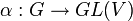$\alpha:G \to GL(V)$ (viz,to the general linear group) where the images of elements of$g$ are ambiguous upto scalar multiples, and such that$\alpha(gh) = \alpha(g)\alpha(h)$ upto a scalar multiple.

if we let$f: G \times G \to k^*$ be the function such that: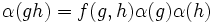$\alpha(gh) = f(g,h)\alpha(g)\alpha(h)$

then we say that$\alpha$ is a$f$-representation.

Two projective representations$\alpha_1: G \to GL(V_1)$ and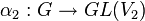$\alpha_2:G \to GL(V_2)$ over a field$k$ are termed projectively equivalent if there exists a vector space isomorphism$F:V_1 \to V_2$ and a function (not necessarily a homomorphism)$\theta:G \to k^\times$ such that for every$g \in G$ and$v\in V$:$F(\alpha_1(g) \cdot v) = \theta(g)(\alpha_2(g) \cdot F(v))$

In other words, they differ by a scalar multiplication combined with a change-of-basis isomorphism.

## Facts

### Linear representations give projective representations

Every linear representation$G \to GL(V)$ gives rise to a projective representation,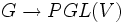$G \to PGL(V)$, simply by composing the given representation with the quotient map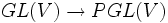$GL(V) \to PGL(V)$ (which involves quotienting out by the center). However, not every projective representation arises from a linear representation.

However, it is very much possible that different linear representations descend to the same projective representation. The following is in fact true:

Two linear representations are projectively equivalent if and only if one of them can be obtained from the other via multiplication by a one-dimensional representation.

In particular, all the one-dimensional representations are projectively equivalent to each other.

### Projective representation gives a 2-cocycle

Let$\alpha$ be a projective representation. Then we can associate to it a 2-cocycle such that:$\alpha(gh) = f(g,h)\alpha(g)\alpha(h)$

By the assumptions for a projective representation, this turns out to be a 2-cocycle from$G$ to$k^*$.

It turns out that projectively equivalent projective representations give 2-cocycles that differ multiplicatively by a 2-coboundary. Thus, any projective representation up to projective equivalence defines an element of the second cohomology group for trivial group action$H^2(G,k^\ast)$.

### When is a projective representation equivalent to a linear representation?

A projective representation is projectively equivalent to a linear representation iff the 2-cocycle associated to it is a 2-coboundary. In particular, this means that if$H^2(G,k^*)$ (the second cohomology group) is trivial, any projective representation is projectively equivalent to a linear representation.

When$k = \mathbb{C}$, this is the same as the assertion that the group has trivial Schur multiplier (or is Schur-trivial).

In general, any projective representation of the group gives rise to a linear representation of its Schur covering group.